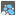## Question related to missionLoading Cargo

I really need some help here because i'm really in a dead end... I have managed to pass the first five assertions and furthermore pass two more examples that were reported to produce an error but now i am really in a place where i don't know what to do... I believe that my problem is that i haven't figured out yet a solid algorithm which will cover all the cases...

The block of code below is the final form of my loading cargo script with the extra assertions, the final is the one that returns an error...

Any help will be truly appreciated...

```    import math
def checkio(data):
N=sum(data)
a=[]
b=[]
c={}
j=0
maxLenNum=0

for i in data:
if len(str(i))>maxLenNum:
maxLenNum=len(str(i))

data.sort(reverse=True)

if (N % 2 == 0):
if (len(data) % 2 == 0):
for i in data:
if (sum(a) < (N/2)):
a.append(i)
else:
b.append(i)
else:
for i in data:
c[j]=i
j=j+1

if maxLenNum == 1:
for key in c:
if sum(a) < N/2:
a.append(c[key])
else:
b.append(c[key])
else:
for key in c:
if key%2 == 0:
a.append(c[key])
else:
b.append(c[key])

if sum(a)>sum(b):
minA=min(a)
a.pop(a.index(minA))
b.append(minA)
elif sum(a)<sum(b):
minB=min(b)
b.pop(b.index(minB))
a.append(minB)

else:
if (len(data) != len(set(data))):
for i in data:
c[j]=i
j=j+1
for key in c:
if key%2 == 0:
a.append(c[key])
else:
b.append(c[key])
if (len(data)%2 ==0):
mina=min(a)
minb=min(b)
a.pop(a.index(mina))
a.append(minb)
b.pop(a.index(minb))
b.append(mina)

else:
for i in data:
if ((sum(a) < sum(b)) or (len(a)==0 and len(b)==0)):
a.append(i)
else:
b.append(i)
return abs(sum(a)-sum(b))

if __name__ == '__main__':
assert checkio([10, 10]) == 0, "1st example"
assert checkio() == 10, "2nd example"
assert checkio([5, 8, 13, 27, 14]) == 3, "3rd example"
assert checkio([5, 5, 6, 5]) == 1, "4th example"
assert checkio([12, 30, 30, 32, 42, 49]) == 9, "5th example"
assert checkio([1, 1, 1, 3]) == 0, "6th example"
assert checkio([5,10,5,10,1]) == 1, "7th example"
assert checkio([33,5,10,19,35,16,10]) == 0, "8th example"
assert checkio([9,9,7,6,5]) == 0, "9th example"
assert checkio([25,43,8,8,31,1,17,7,3]) == 1, "10th example"
```